Back to Riemann, Part 7

Before expanding the zeta function to the complex plane, I want to talk about Euler a little more.

Recall the zeta function:

z(x) = 1 + 1/2^x + 1/3^x + 1/4^x…

Euler assumed that x would be small, for -1 < x < 1.

He then defined a function M(x), where there are infinite terms,

M(x) = 1 + x + x^2 + x^3 + x^4…

Then, he multiplied both sides by x,

x*m(x) = x + x^2 + x^3 + x^4 + x^5…

And subtracted both sides, M(x) – x*M(x):

M(x) – x*M(x) = 1 + x – x + x^2 – x^2 + x^3 – x^3 + x^4 – x^4…
M(x) – x*M(x) = 1
M(x)(1 – x) = 1
M(x) = 1/(1 – x)

Ex. M(0.5) = 1/(1 – 0.5) = 2

He followed this up by applying the same approach for x > 1 for the zeta function.

z(x) = 1 + 1/2^x + 1/3^x + 1/4^x…

1/2^x * z(x) = 1/2^x + 1/4^x + 1/6^x + 1/8^x…

1/2^x * z(x) – z(x) = -1 +1/2^x – 1/2^x – 1/^3^x + 1/4^x – 1/4^x – 1/5^x…

z(x)(1 – 1/2^x) = 1 + 1/3^x + 1/5^x + 1/7^x…

This removes all multiples of 2 from the right hand side. Repeating for 1/3^x:

z(x)(1 – 1/2^x)(1 – 1/3^x) = 1 + 1/5^x + 1/7^x + 1/11^x…

Removes all multiples of 2 and 3, etc.

Solving for z(x),

z(x) = (1/(1-1/2^x))*(1/(1-1/3^x))*(1/(1-1/5^x))*(1/(1-1/7^x))…

This is called Euler’s product formula, and it just contains terms with prime numbers in the denominators. And what this does is directly tie the zeta function to the prime numbers. Unfortunately, we’re still faced with the problem of not knowing the distribution of those prime numbers, or what the next one in a very long sequence will be. But, at least now we have a clear connection to the zeta function and the primes.

This probably a good place to talk about zeroes.

For certain polynomials, you can determine their function if you know where they cross the x-axis, and their value when x=0.

Example from the der Veen and de Craats book:
Say you have p(x), with p(2) = p(3) = 0, and p(0) = 1

This is a parabola pointing up, and can be written as:
p(x) = (1 – x/2)*(1 – x/3)
p(x) = 1 – (x*5)/6 + (x^2)/6

Expanding this function for zeroes at all integers 1 through 10,

p(x) = (1 – x/1)(1 – x/2)(1 – x/3)…(1 – x/10)

A simple question now: What’s the coefficient for x?

Answer, this is the component where we just have the a*x term, which is:
x/1 + x/2 + x/3… + x/10
= x*(1 + 1/2 + 1/3 + … + 1/10)

So, Euler assumed that S(x) = sin(pi*x)/pi*x
was a polynomial of infinite degree, with zeroes at …-4, -3, -2, -1, 1, 2, 3, 4…
Basically, all real integers excluding 0. And S(0) = 1.

Therefore,
S(x) = (1 – x/1)(1 – x/(-1))(1 – x/2)(1 – x(-2))(1 – x/3)(1 – x/(-3))…

Because (1 – x/3)(1 – x/(-3)) = (1 – ((x^2)/(3^2)) (1 minus x-squared divided by 3-squared),

S(x) = sin(pi*x)/pi*x = (1 – (x^2)/(1^2))(1 – (x^2)/(2^2))(1 – (x^2)/(3^2))…

BUT,
If we use the geometric expansion for sin(x),
sin(x) = x – x^3/3! + x^5/5! – x^7/7!…

And substitute pi*x for x,
sin(pi*x) = pi*x – (pi^3)*(x^3)/(3!) + (pi^5)*(x^5)/(5!) – (pi^7)*(x^7)/(7!)…

And divide both sides by pi*x,

sin(pi*x)/(pi*x) = 1 – (pi^2)*(x^2)/(3!) + (pi^4)*(x^4)/(5!) – (pi^6)*(x^6)/7!…

Euler found that the coefficient for x^2 is -(pi^2)/(3!) = -(pi^2)/6.

And, taking the above polynomial for finding the zeroes of S(x), the same coefficient for x^2 is
-x^2(1/1^2 + 1/2^2 + 1/3^2 + 1/4^2)
or,
-1/1^2 – 1/2^2 – 1/3^2 – 1/4^2…

And this is the zeta function for minus zeta(2). That is, the above zeta function, negative, with x=2.

From this Euler concluded that zeta(2) = pi^2/6

der Veen and de Crats say that this isn’t a really rigorous proof, but the end result is correct.
But, by using the same method above, Euler calculated zeta(x) for all even positive integers.
The odd positive integers are much harder, and they don’t work out to be as pretty as for the first few even integers. A little more information on them can be found in the wiki article.(Image taken from the wiki page.)

The even numbers can also be found using the Bernoulli formula, where B2n is the 2nth Bernoulli number. (Note that because the odd-numbered Bernoulli values, excluding the first 1, equal zero, the above formula doesn’t work for the odd-numbered Zeta integers.)

Anyway. The above approaches let us get the zeta(x) values for the positive integer values for x, which are all non-zero.

Back to Riemann, Part 6

In the last entry, I introduced the prime number counting function, pi(x), and one equation used for approximating it. Remember that pi(x) actually does count the number of prime numbers less than or equal to “x”. But, this is a very slow process, and it doesn’t tell us anything about how the primes are distributed across the real axis. The approximation, x/ln(x), at the least, comes close to pi(x) for sufficiently large values of x. And, the relative error, as given by (pi(x) – x/ln(x))/x goes to zero for large x (there’s still an error, but it’s comparatively small given how many prime numbers we’re really talking about).

Now, we can define any kind of counting function that we like, such as the number of even numbers less than or equal to “x”, the number of odd numbers, or the number of cups of coffee I drink each day of the week. And, if we can plot those numbers, we can try to find an approximation for them in order to predict future events. In cases like with the even numbers, the approximation is going to be 100% accurate (T(x) = x/2, truncated), and the error is going to be 0 for all x. For a function Tc() for counting cups of coffee per day, Tc(x)=Number_of_Days will have an error of +/- 2 depending on how tired I am, but the relative error Tc(x)/x will go to zero for large “x” (that is, for a period of 3 weeks or longer), because I normally only drink one cup a day.

The Russian mathematician Pafnuti Chebyshev, in about 1850, introduced a new logarithmic prime counting function he called psi(x). He defined psi(x) to be the number of prime powers less than or equal to x, but he weighted them based on how frequently they occurred within the range.

psi(x) = T2*ln(2) + T3*ln(3) + T5*ln(5) + T7*ln(7) + T11*ln(11)…

In this case, the T components are counting functions for each prime number. Say we want to look at psi(100).

The powers of 2 less than 100 are: 2, 4, 8, 16, 32 and 64, so T2 = 6.
The powers of 3 less than 100 are: 3, 9, 27 and 81, so T3 = 4.
The powers of 5 less than 100 are: 5 and 25, so T5 = 2.
The powers of 7 less than 100 are: 7 and 49, so T7 = 2.
The powers of all other primes up to 97 occur once only.
After that, T=0 for all primes > 100.

psi(100) = 6*ln(2) + 4*ln(3) + 2*ln(5) + 2*ln(7) + 1*ln(11) + 1*ln(13)…
= 94

The idea here is that although it’s not practical to count the prime powers, this approximation goes to “x” for large values of “x”, and the relative error, (psi(x) – x)/x goes to zero. (Ex.: x = 100, and psi(100) = 94. Rel error = (100-94)/100 = 0.06)

And, psi(x) is going to have pi(x) terms. pi(100) = 25, so there will be 25 terms in psi(100), from T2*ln(2) up to T97*ln(97). psi(x) can be approximated by pi(x)*ln(x), and this is then further proof that pi(x) can be approximated by x/ln(x).

The take away here is that psi(x) is based on the prime numbers themselves, while pi(x) is tied specifically to x and ln(x).

Ok, so let’s go back to the zeta function,
z(x) = 1 + 1/2^x + 1/3^x + 1/4^x…

Euler used a modified form of the zeta function, defined over the range -1 < x < 1, as follows:
M(x) = 1 + x + x^2 + x^3 + x^4…

Now, if you multiply both sides by “x”,
x*M(x) = x + x^2 + x^3 + x^4 + x^5…

And then subtract equation 1 from equation 2, and group the M(x) terms,
M(x) – x*M(x) = 1 + x – x + x^2 – x^2 + x^3 – x^3…

Meuler(x) = 1/(1 – x) for -1 < x < 1 If we plug 2 into the original equation, Moriginal(2) = 1 + 2^2 + 3^2 + 4^2… the series goes to infinity. But, using the identity, Meuler(2) = 1/(1 – 2) = -1, the equation converges. We can use a similar approach with the zeta function. Recall that in its original form, z(x) converges only for x > 1 (so its domain is 1 < x < infinity). We can extend this domain as follows by defining eta(), as invented by Gustav Dirichlet (1805-1859):

eta(x) = 1 – 1/2^x + 1/3^x – 1/4^x + 1/5^x…

The minus signs cause the terms to cancel out faster, and the series then grows more slowly for positive x, even when x < 1.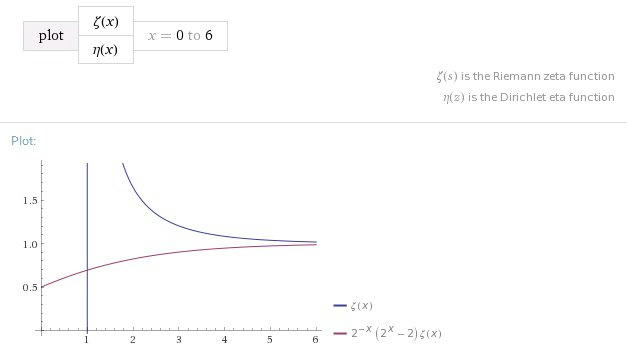(Coverage of domains by zeta(x) (top line) and eta(x) (bottom line).)

If we return to the geometric series (which I used to show that e^x is directly related to cos(x) and sin(x)), we get another equation, specifically:

ln(x+1) = x – x^2/2 + x^3/3 – x^4/4 + x^5/5…

Plugging in x = 1,

ln(2) = 1 – 1/2 + 1/3 – 1/4 + 1/5…
eta(1) = 1 – 1/2 + 1/3 – 1/4 + 1/5…
So that eta(1) = ln(2) = 0.693…

and, eta(0.5) = 0.6049…

Ok, what we want is the connection between zeta and eta. First, subtract both sides:

z(x) = 1 + 1/2^x + 1/3^x + 1/4^x…
e(x) = 1 – 1/2^x + 1/3^x – 1/4^x + 1/5^x…

z(x) – e(x) = (1-1) + (1/2^x + 1/2^x) + (1/3^x – 1/3^x)…
z(x) – e(x) = 2/2^x + 2/4^x + 2/6^x…

Factor out 2/2^x = 2^(1-x)

z(x) – e(x) = 2^(1-x) * (1 + 1/x^2 + 1/x^3 + 1/x^4…)
z(x) – e(x) = 2^(1-x) * z(x)

e(x) = z(x) * (1 – 2^(1-x)), or
z(x) = e(x) / (1 – 2^(1-x))

What this means is that, while we can’t find zeta(x) for x between 0 and 1, we can find eta(x) for that range. And, using the conversion formula here, we can map eta(x) to zeta(x) for this same range.

Because eta(x) converges for all real numbers x, with the exception of 1, we can define the behavior of the zeta function for 0 < x < 1. This extends the domain of the zeta function beyond its original 1 < x < infinity.

As an example, eta(0.5) was shown to be 0.6049… above. So,
zeta(0.5) = 0.6049 / (1 – sqrt(2)) = -1.46035…

And,
eta(0) = 0.5
zeta(0) = 0.5 / (-1) = -0.5

The next step is to expand the domain for the zeta function to the complex plane.

Back to Riemann, Part 5

So far, I’ve covered exponents, i, e, ln() and series expansions.

n^x is a continuous line from minus to plus infinity if n is not 0 or 1, and x is a real number.

If n = e, when x = a + bi, then e^x = e^(a+bi) = (e^a) * (cos(b) + i*sin(b))

And, if a=0 and b = pi (3.14159),
e^i*pi = -1

making e^bi the function for drawing the unit circle.

Then, ln(), the natural logarithm, is just the inverse function for e^x.

I kind of jumped from n^x, for any value of n, to e^x. We can convert between them if we want to. If j = n – e, then n^x = (j + e)^x.

And, logb(x) for any base “b” is = ln(x) / ln(b).

Again, why use “e” and ln()? Because they’re easy to work with, they have special properties that other numbers don’t have, such as e^i*pi being on the unit circle, and we can always convert between them and base 10 numbers if we have to.

Why use series expansions? Well, that’s what the zeta function is (1 + 1/2^s + 1/3^s + 1/4^s + 1/5^s…), and some functions that are harder to work with in one form (e^(a+bi)) can be easier to work with in another (e^x = 1 + x + x^2/2! + x^3/3! + x^4/4! + x^5/5!…)

Ok, what’s the point?
So far, I’ve just wanted to lay down the groundwork. It’s now time to look at how everything started, to lead up to the Riemann Hypothesis.

We begin by trying to count the number of prime numbers below a particular limit.

A number is prime if its only factors are itself, and 1. Because 1 is a factor of all other numbers, it is not included in the list of primes. That means that 2, 3, 5 and 7 are the only primes less than or equal to 10. 4 is not prime because its factors are 1, 2 and 4. 6 is not prime because its factors are 1, 2, 3 and 6. 8 is not prime because its factors are 1, 2, 4 and 8. 9 is not prime because its factors are 1, 3 and 9.

We can define a counting function, usually given as:pronounced “pi”, but is not to be confused with the constant 3.14159. This function counts the number of primes from 2 to x.

pi(10)  = 4
pi(100) = 25
pi(1000) = 168

pi() as a function doesn’t have an equation to it, per se. At least, not just yet. It’s just a matter of sitting down and checking whether every number between 2 and 1000 is prime or non-prime, and then counting up all the ones that are prime.(Plots here obtained from Wolfram Alpha. Above plot is for pi(10,000).)

What’s important here is to note that the plot of pi() from 2 to 10,000 is not linear. If we want to find some way of getting close at guessing the correct answers for how many primes there are between 100,000 and 199,999, we can’t use an expression in the form of y = n * x, or y = x^n, because they’re going to deviate too much from the real answer given by pi().

We could try using x * 0.125, or x^0.773, which look like they might be getting close to pi() in the range 0 to 10,000: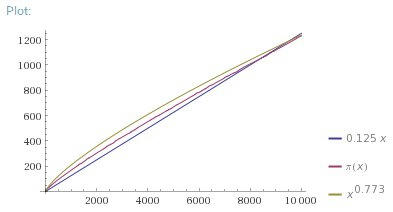But, if we expand the range (referred to as changing the domain of the function) to 0 to 10,000,000, both approximations obviously fail.Instead, it turns out that x/ln(x) works a lot better over the larger domains, and

pi(x) ~ x/ln(x)

is known as the Prime Number Theorem, where the squiggly means that the error between pi(x) and x/ln(x) goes to zero as x goes to infinity.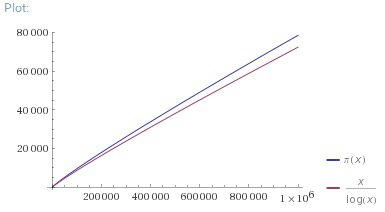Remember, pi(x) actually does count the number of primes less than or equal to x, so that’s the truly correct value. But, hand-counting the primes is time consuming, so we want to find an equation that gives us a close approximate answer, and we use x/ln(x) for that.

How do we get the error between reality and the approximation?

error = pi(x) – x/ln(x)

And, we can introduce the idea of “a relative error”, which is (pi(x) – x/ln(x)) / x.

Or, the difference between the counting function pi() and the approximation x/ln(x), divided by x. If the relative error goes to 0 as x goes to infinity, then the number of primes below x is about x/ln(x), for large x.

What this means is that one of the closest methods we have, at the moment, for predicting the distribution of prime numbers is the formula x/ln(x). We’re going to try to find something better.(Relative error for pi(x) and x/ln(x) from 0 to 1 million.)

Very quickly, one reason people care about prime numbers is that they’re used extensively in securing computer communications and data encryption. If you pick a very large number (150 digits) that is the product of 2 prime numbers, then you can make that number public, and it will be very difficult to determine which two prime numbers make up its factors. That is, it’s easy to test whether a random number is prime, but it’s much, much harder computationally to find the factors of that number. There can be billions of prime numbers that are 100 digits long, and the odds of randomly giving two people the exact same key are very near zero. So, for the moment, public key encryption is considered pretty secure, if you use long enough numbers. However, if there were some way of predicting the distribution of prime numbers exactly, it would become much easier to crack public key systems.

Back to Riemann, Part 4

Ok, let’s get back on topic for e^bi.

Or, maybe not.
I want to look at one more way to calculate e.

The zeta function is generally written as:

z(x) = 1 + 1/2^x + 1/3^x + 1/4^x + 1/5^x…

on into infinity, where x can have the value of a + bi across the complex plane.

If we set x = 1, we get the geometric series, AKA the Harmonic Series:

z(1) = 1 + 1/2 + 1/3 + 1/4 + 1/5…

Which doesn’t converge. It just goes to infinity kind of slowly.

Setting x = 2, however, gives us the series:

z(2) = 1 + 1/2^2 + 1/3^2 + 1/4^2 + 1/5^2…
z(2) = pi^2 / 6 = 1.6449…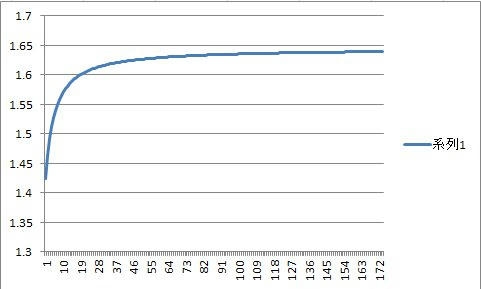I ran the above series in Excel, to 1/177^2, and got 1.6393, which is pretty close.

z(3) = 1 + 1/2^3 + 1/3^3 + 1/4^3 + 1/5^3…
Which approaches 1.202041
z(4) = pi^4/90 = 1.0823…

So, what this says is that the zeta function z(x) converges for x > 1, but it doesn’t converge to 0 (it converges to 1 for large values of x).

There’s also a series that converges to e^x:

e^x = 1 + x + x^2/2! + x^3/3! + x^4/4! + x^5/5!…

Where “!” is the symbol for factorial, or for multiplying the integers from 1 to n together.

2! = 1*2 —– = 2
3! = 1*2*3 — = 6
4! = 1*2*3*4 – = 24
5! = 1*2*3*4*5 = 120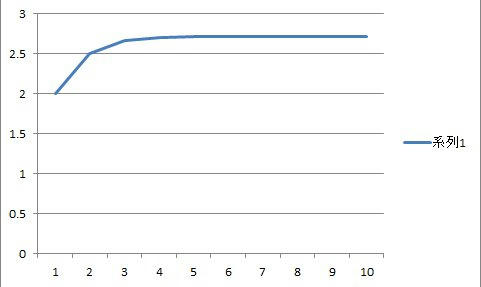(After only 10 terms, the series is already at 2.71828, and the curve looks flat.)

In the last blog entry, I mentioned that taking the derivative of f(x) = e^x => f'(x) = e^x.
We can prove this by taking the derivative of the series above:

d/dx e^x = d/dx (1 + x + x^2/2! + x^3/3! + x^4/4! + x^5/5!…)

Recall that if f(x) = x^b, then
d/dx f(x) = b * x^(b-1)

Note also that d/dx of a constant = 0.
We can take the derivatives of each term in the series separately, as in:

d/dx (1) = 0
d/dx (x) = 1*x^0 = 1
d/dx (x^2) = 2*x
d/dx (x^3) = 3*x^2
d/dx (x^4) = 4*x^3
d/dx (x^5) = 5*x^4
etc.

Plugging the terms back into f'(x),
f'(x) = 0 + 1 + 2*x/(1*2) + 3*x^2/1*2*3 + 4*x^3/1*2*3*4…
f'(x) = 1 + x + x^2/2! + x^3/3! + x^4/4! + x^5/5!…
f'(x) = e^x

What’s interesting about this infinite series approach is that it can also be used to find values for sin(x) and cos(x)

sin(x) = x – x^3/3! + x^5/5! – x^7/7! + x^9/9!…
cos(x) = 1 – x^2/2! + x^4/4! – x^6/6! + x^8/8!…

Now, what happens if we play with e^iy?

e^iy = 1 + iy + (iy)^2/2! + (iy)^3/3! +(iy)^4/4! + (iy)^5/5!…
e^iy = 1 + iy – y^2/2! + iy^3/3! + y^4/4! + iy^5/5! – y^6/6!…

If we group the terms with and without i components together,
e^iy = (1 – y^2/2! + y^4/4! – y^6/6!…) + i(y – y^3/3! + y^5/5!…)

Look at the series expansions for sin() and cos() above.

e^iy = cos(y) + i*sin(y)

And, if we pick y = pi
e^i*pi = cos(pi) + i*sin(pi) = -1 + 0 = -1

What’s this tell us? That e^iy defines the unit circle.

Therefore, e^x, where x = a+bi, gives us e^(a+bi) = (e^a) * (e^bi)
= e^a * (cos(b) + i*sin(b))
(if a=0, e^a  = 1)(The unit circle as a function of e^bi, which we already saw in Riemann Prose part 7.)

Hanayama Puzzle – ChainHanayama is a Japanese producer of metal puzzles. I received this one, called “Chain,” from the U.S. as a Christmas present. On a difficulty ranking of 1 to 6, this one’s rated a 6. But, that’s a bit misleading. If you look at the photo closely, you may figure out the solution visually. This is because there’s an “easy problem” and a “hard problem”.I’ve written before about how I view wire puzzles as a form of magic trick. A lot of the design revolves around misdirection. If you try to attack the puzzle in what appears to be the more obvious solution, you’re going to fail horribly. You have to sit back, think, and ask yourself, why is this bend where it is? This feature? Why does one piece have this feature, and the others don’t?After about an hour, I decided to check youtube to see if anyone had posted videos there. The answer was that they had, so I watched the first few seconds of one to get a hint, then went back to the puzzle. The hint didn’t help, so I checked a second video. That didn’t help either. The reason was that the videos were showing the easy approach of the puzzle, and the one I had was assembled for the hard approach from the factory.

The box has a challenge printed on it – “You may be able to take this puzzle apart, but can you put it back together?” Eventually, I realized what the trick was and I could pull the three links apart. Putting the puzzle back together, I quickly verified that there really are 2 variants – the easy approach and the hard one. The interesting thing is that there’s a trick to assembling the hard approach, also. There are many ways to do it wrong, but only one way to do it right, which isn’t intuitive.

I brought Chain with me to a party, and I passed it around to about 10 people there. Even in the easy approach, only one person got it right. I had to show the others how to take it apart, which made them really happy. Then I pulled the old stage magic prank of assembling the puzzle with the hard approach without telling them, and watched their faces as they discovered that even if you know the solution, it still doesn’t help.

If you like puzzles, the Hanamaya collection is challenging, cheap for their weight, and very durable. Recommended to puzzle lovers. (As a note, I can take Chain apart in either approach and reassemble it within 30 seconds now.)